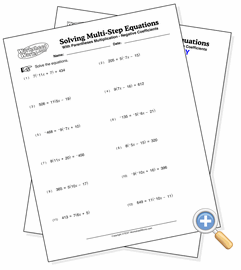# With Parentheses and Multiplication

## Solving Multi-Step EquationsSolve equations involving parentheses

These equations contain values multiplied through parentheses. They can be solved by either dividing both sides of the equation by the value or by first distributing the value to the terms inside the parentheses. Our solutions show the first approach, but they can be used to demonstrate distributed multiplication too. In whichever way they are solved, all problems resolve to integers.